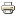# Probabilistic voting equilibria under nonlinear candidate payoffsArticle
Author/s:
Alexei Zakharov
Journal of Theoretical Politics
Year:
2012
Journal pages:
235-247
The “mean voter theorem” implies that candidates should choose identical policy positions in a two-candidate race if voting is probabilistic. This result is in fact an artifact of the assumption that the candidates maximize expected vote share or probability of win, which is not true for many real-world elections. In this paper I analyze a probabilistic voting model in which the candidates have preferences other than the maximization of the expected number of votes or the probability of win maximization. I derive the comparative statics for two voters and one-dimensional policy space. Each voter cares about both the policy platform and the identity of the candidate. It is shown that an increase in the value of exactly one vote causes each candidate to choose a position closer to that of its partisan voter. Numeric computation of equilibria show that these results can be generalized to three or more voters. The results imply that the nonlinearity and nonsymmetry of payoﬀs can aﬀect the policy positions of the candidates.
Developed by Paolo Gittoi